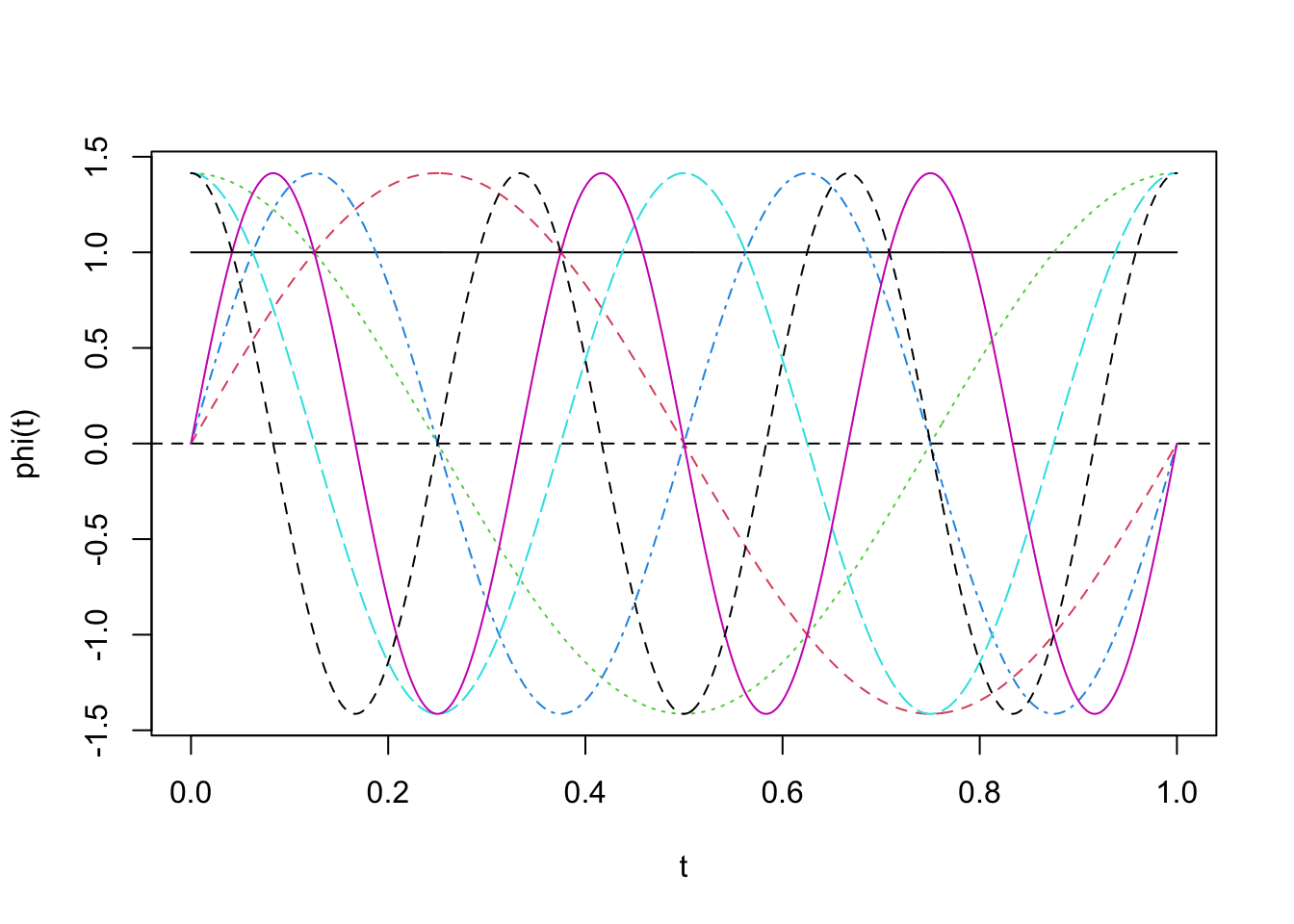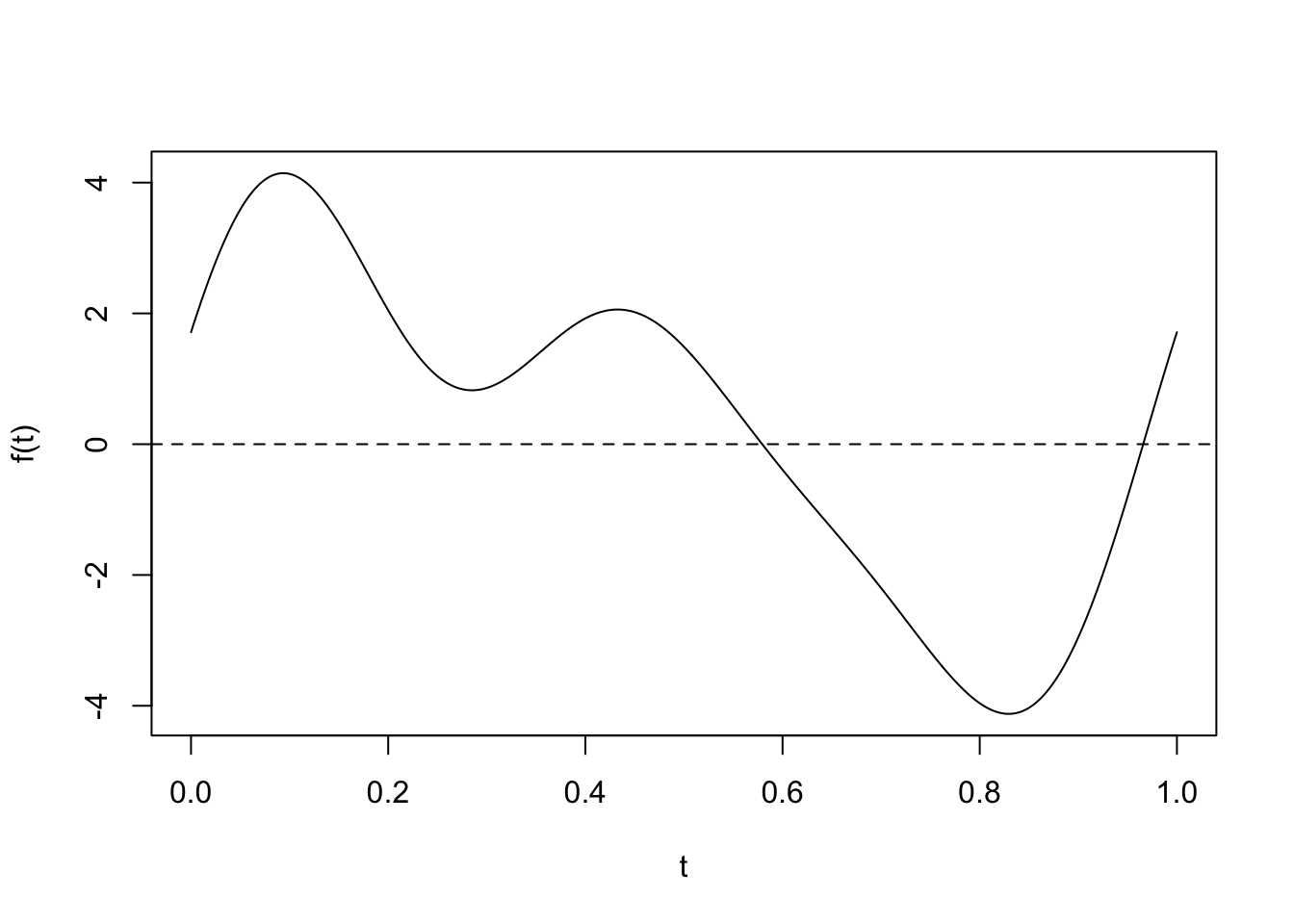library(fda)
#>
#> Attaching package: 'fda'
#> The following object is masked from 'package:graphics':
#>
#>     matplot

# The Basics of Basis Functions

## What is a basis function?

Here’s the problem. We want a mathematical description of a curve or any other data distributed over space, time, and other types of continuum. Flexibility is a central issue since we usually cannot say in advance how complex the curve will be, or specify certain of its characteristics. We don’t have the time or patience to search some handbook of known functions for one that looks like what we want to study, either. Moreover, however it is that we design our mathematical function, we will want to do any computation that is needed to fit the data quickly and with a minimum of programming.

We need, therefore, a set of basic functional building blocks that can be stacked on top of one another so as to have the features that we need. Mathematical Lego, Meccano, Erector, Tinker Toy, or whatever, in other words. Since this is mathematics, we use the symbol $$\phi_k(t)$$ to stand for the $$k$$th function in our toy box, and we call this the $$k$$th basis function.

By “stacking” in mathematics we mean adding things, possibly after multiplying each of them by its own constant. So here is how we will construct a function $$f(t)$$ using $$K$$ of these blocks: $f(t) = c_1 \phi_1(t) + c_2 \phi_2(t) + \ldots + c_K \phi_K(t)$

In math-speak, this is a “linear combination”. The construction of an actual function then becomes a matter of assigning values to the $$Κ$$ constants $$a_k$$.

## What are some examples of commonly used basis functions?

### Powers of $$t$$

There are many ways to put together a kit of basis functions. The oldest system consists of the powers of $$t$$, that is, $$1, t, t_2, ... , t_k$$. Linear combinations of these functions are called polynomials.

### Fourier basis functions: 1, sines and cosines

A system developed in early nineteenth century France is the Fourier basis, consisting of $$1$$ and a series of pairs of sines and cosines of increasing frequency; that is, $$\phi_1(t) = 1, \phi_2(t) = \sin(\omega t), \phi_3(t) = \cos(\omega t), \phi_4(t) = \sin(2 \omega t), \phi_5(t) = \cos(2 \omega t), \ldots$$.

The constant $$\omega$$ plays an important role: functions constructed with this system repeat themselves each time $$t$$ increases by $$2 \pi / \omega$$ units. Consequently, we tend to use this basis for periodic functions.

This code shows the first seven basis functions in the series with a period of one unit.#>  "done"

Here we set up a single function by using a random set of coefficient values. Note that the curve has a complex shape, but does have the same value at the beginning and end. We automatically plot a dashed horizontal line in order see where the function has value zero.

coefs <- matrix(rnorm(7),7,1)
onefourier.fd <- fd(coefs, fourier.basis)
plot(onefourier.fd, xlab="t", ylab="f(t)")#>  "done"

### Localized polynomials: splines

Polynomials, though simple and familiar, are actually not that flexible, and consequently they have been more or less replaced by a basis system called the spline basis system. Splines, although related to polynomials systems, require some further explanation, and we have some special notes on them in this website. However, you can have a preliminary look at spline basis functions in the following figure.

nbasis <- 8
rangeval <- c(0,10)
spline.basis <- create.bspline.basis(rangeval, nbasis)
coefs <- diag(rep(1,8))
spline.fd <- fd(coefs, spline.basis)
plot(spline.fd, xlab = "t", ylab = "phi(t)")